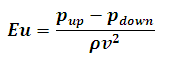# Euler Number Calculator

This Calctown Calculator calculates the Euler number used in fluid flow calculations used to characterize losses in the flow.

Pa
Pa
kg/m3
m/s

#### Resultwhere

Eu = Euler number

pup = upstream pressure

pdown = downstream pressure

ρ = density of fluid

v = velocity of the fluid